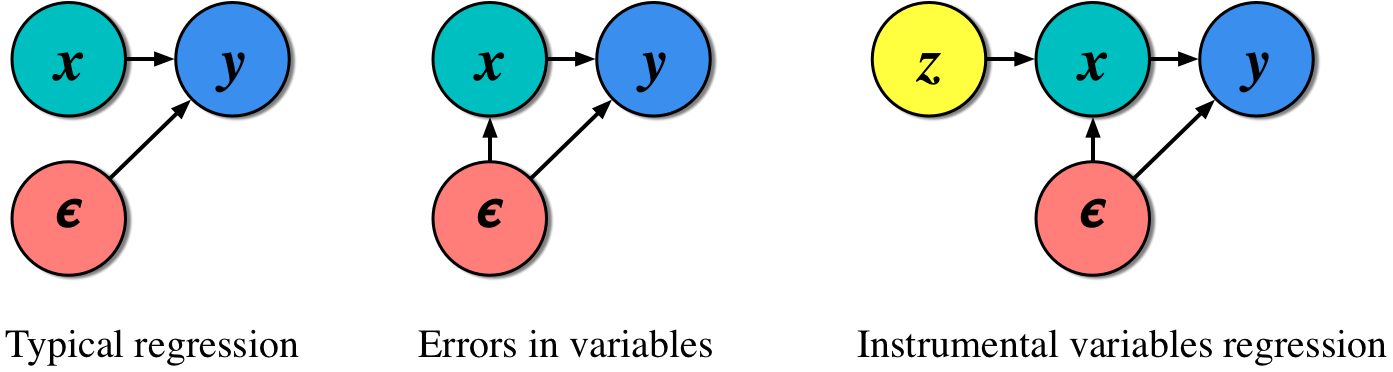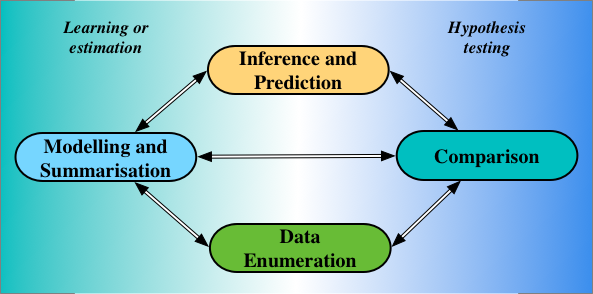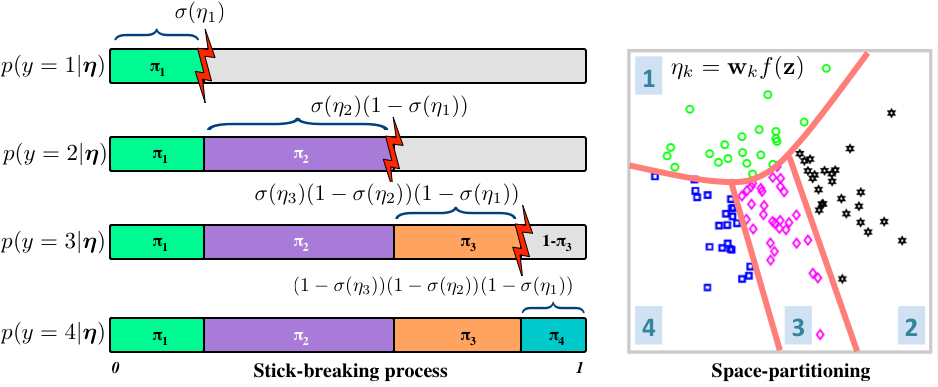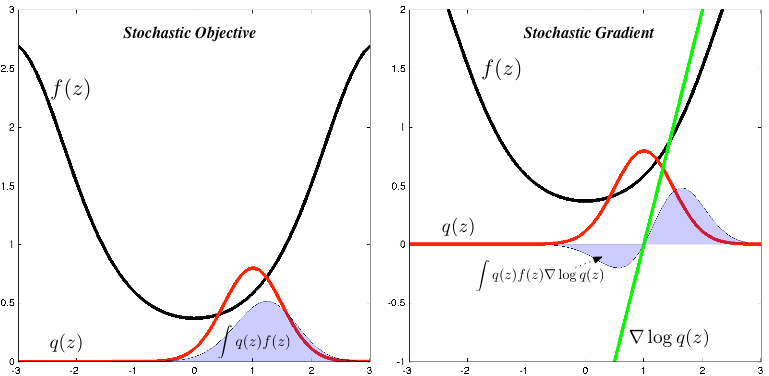# Machine Learning Trick of the Day (8): Instrumental Thinking

· Read in · 1499 words · All posts in series · [dropcap]This[/dropcap] trick is unlike the others we've conjured. It will not reveal a clever manipulation of a probability or an integral or a derivative, or produce a code … Continue reading Machine Learning Trick of the Day (8): Instrumental Thinking# Machine Learning Trick of the Day (7): Density Ratio Trick

· Read in · 1481 words · All posts in series · [dropcap]A[/dropcap] probability on its own is often an uninteresting thing. But when we can compare probabilities, that is when their full splendour is revealed. By comparing probabilities we … Continue reading Machine Learning Trick of the Day (7): Density Ratio Trick# Machine Learning Trick of the Day (6): Tricks with Sticks

· Read in  · Our first encounters with probability are often through a collection of simple games. The games of probability are played with coins, dice, cards, balls and urns, and sticks and strings. Using these games, we built an intuition that … Continue reading Machine Learning Trick of the Day (6): Tricks with Sticks# Machine Learning Trick of the Day (5): Log Derivative Trick

Machine learning involves manipulating probabilities. These probabilities are most often represented as normalised-probabilities or as log-probabilities. An ability to shrewdly alternate between these two representations is a vital step towards strengthening the probabilistic dexterity we need to solve modern machine learning problems. Today's trick, the log derivative trick, helps … Continue reading Machine Learning Trick of the Day (5): Log Derivative Trick# Machine Learning Trick of the Day (4): Reparameterisation Tricks

Our ability to rewrite statistical problems in an equivalent but different form, to reparameterise them, is one of the most general-purpose tools we have in mathematical statistics. We used reparameterisation in all the tricks we explored in this series so far: trick 1 re-expressed a … Continue reading Machine Learning Trick of the Day (4): Reparameterisation Tricks# Machine Learning Trick of the Day (3): Hutchinson's Trick

Hutchinson's estimator [cite key='hutchinson1990stochastic'] is a simple way to obtain a stochastic estimate of the trace of a matrix. This is a simple trick that uses randomisation to transform the algebraic problem of computing the trace into the statistical problem of … Continue reading Machine Learning Trick of the Day (3): Hutchinson's Trick# Machine Learning Trick of the Day (2): Gaussian Integral Trick

Today's trick, the Gaussian integral trick, is one that allows us to re-express a (potentially troublesome) function in an alternative form, in particular, as an integral of a Gaussian against another function — integrals against a Gaussian turn out not to be too troublesome … Continue reading Machine Learning Trick of the Day (2): Gaussian Integral Trick

# Machine Learning Trick of the Day (1): Replica Trick

'Tricks' of all sorts are used throughout machine learning, in both research and in production settings. These tricks allow us to address many different types of data analysis problems, being roughly of either an analytical, statistical, algorithmic, or numerical flavour. Today's trick is in the analytical class and comes to us from statistical physics: the popular Replica trick. The replica trick [cite key="engel2001statistical"][cite key="sharp2011effective"][cite key="opper1995statistical"] is used for analytical computation of log-normalising constants (or log-partition functions). More formally, the replica trick provides one of the tools needed for a replica analysis of a probabilistic model — a theoretical analysis of the the properties and expected behaviour of a model. Replica … Continue reading Machine Learning Trick of the Day (1): Replica Trick